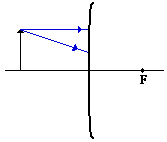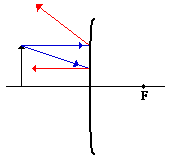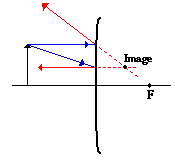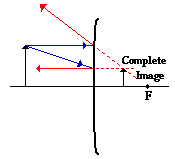Rules of ray tracing for convex mirror

• Any incident ray traveling parallel to the principal axis on the way to a convex mirror will reflect in such a manner that its extension will pass through the focal point.
• Any incident ray traveling towards a convex mirror such that its extension passes through the focal point will reflect and travel parallel to the principal axis.
• Rules provided by Physics Classroom

The method of drawing ray diagrams for convex mirrors is described below.1. Pick a point on the top of the object and draw two incident rays traveling towards the mirror.

Using a straight edge, accurately draw one ray so that it travels towards the focal point on the opposite side of the mirror; this ray will strike the mirror before reaching the focal point; stop the ray at the point of incidence with the mirror. Draw the second ray such that it travels exactly parallel to the principal axis. Place arrowheads upon the rays to indicate their direction of travel.2. Once these incident rays strike the mirror, and then reflected.

The ray that travels towards the focal point will reflect and travel parallel to the principal axis. Use a straight edge to accurately draw its path. The ray that traveled parallel to the principal axis on the way to the mirror will reflect and travel in a direction such that its extension passes through the focal point. Align a straight edge with the point of incidence and the focal point, and draw the second reflected ray. Place arrowheads upon the rays to indicate their direction of travel. The two rays should be diverging upon reflection.3. Locate and mark the image of the top of the object.

The image point of the top of the object is the point where the two reflected rays intersect. Since the two reflected rays are diverging, they must be extended behind the mirror in order to intersect. Using a straight edge, extend each of the rays using dashed lines. Draw the extensions until they intersect. The point of intersection is the image point of the top of the object. Both reflected rays would appear to diverge from this point. If your were to draw a third pair of incident and reflected rays, then the extensions of the third reflected ray would also pass through this point. This is merely the point where all light from the top of the object would appear to diverge from upon reflecting off the mirror. Of course, the rest of the object has an image as well and it can be found by applying the same three steps for another chosen point.4. Repeat the process for the bottom of the object.

The goal of a ray diagram is to determine the location, size, orientation, and type of image that is formed by the convex mirror. Typically, this requires determining where the image of the upper and lower extreme of the object is located and then tracing the entire image. After completing the first three steps, only the image location of the top extreme of the object has been found. Thus, the process must be repeated for the point on the bottom of the object. If the bottom of the object lies upon the principal axis (as it does in this example), then the image of this point will also lie upon the principal axis and be the same distance from the mirror as the image of the top of the object. At this point the complete image can be filled in.

Rules and Steps provided by Physics Classroom

How to solve a Convex Mirror problem mathematically

First things first, lets identify the variables in the problem.

o = object distance, how far the object (in this case the light bulb) is from the mirror.

i = Image distance, very the object appears to be because of the mirror.

f = focal length, a property of the mirror, for convex mirror (because it's virtual) the focal length is negative

h= height of the object

o = 35.5 cm       i = ?      f = -12.2 cm      h= 4.0 cm

Things that students screw up, when solving the mirror equation remember "least common denominator". You simple can't add denominator together.

As for the magnification equation, an important note a negative magnification means a flipped image, positive magnification the image is right side up.

Example Problem

A 4.0-cm tall light bulb is placed a distance of 35.5 cm from a convex mirror having a focal length of -12.2 cm. Determine the image distance and the image size.

Like all problems in physics, begin by the identification of the known information.

 ho = 4.0 cm do = 35.5 cm f = -12.2 cm

Next identify the unknown quantities that you wish to solve for.

 di = ??? hi = ???

To determine the image distance (di), the mirror equation will have to be used. The following lines represent the solution to the image distance; substitutions and algebraic steps are shown.

1/f = 1/do + 1/di

1/(-12.2 cm) = 1/(35.5 cm) + 1/di

-0.0820 cm-1 = 0.0282 cm-1 + 1/di

-0.110 cm-1 = 1/di

 di = -9.08 cm

To determine the image height (hi), the magnification equation is needed. Since three of the four quantities in the equation (disregarding the M) are known, the fourth quantity can be calculated. The solution is shown below.

hi/ho = - di/do

h/(4.0 cm) = - (-9.08 cm)/(35.5 cm)

h= - (4.0 cm) • (-9.08 cm)/(35.5 cm)

 hi = 1.02 cm

Most of this entire page is provided by Physics Classroom